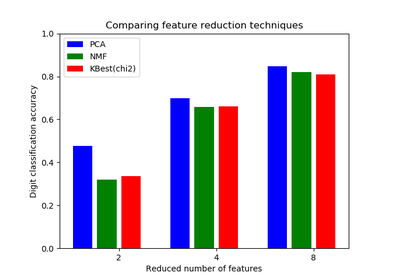# `sklearn.feature_selection`.SelectKBest¶

class `sklearn.feature_selection.``SelectKBest`(score_func=<function f_classif>, k=10)[source]

Select features according to the k highest scores.

Read more in the User Guide.

Parameters: score_func : callable Function taking two arrays X and y, and returning a pair of arrays (scores, pvalues) or a single array with scores. Default is f_classif (see below “See also”). The default function only works with classification tasks. k : int or “all”, optional, default=10 Number of top features to select. The “all” option bypasses selection, for use in a parameter search. scores_ : array-like, shape=(n_features,) Scores of features. pvalues_ : array-like, shape=(n_features,) p-values of feature scores, None if score_func returned only scores.

`f_classif`
ANOVA F-value between label/feature for classification tasks.
`mutual_info_classif`
Mutual information for a discrete target.
`chi2`
Chi-squared stats of non-negative features for classification tasks.
`f_regression`
F-value between label/feature for regression tasks.
`mutual_info_regression`
Mutual information for a continuous target.
`SelectPercentile`
Select features based on percentile of the highest scores.
`SelectFpr`
Select features based on a false positive rate test.
`SelectFdr`
Select features based on an estimated false discovery rate.
`SelectFwe`
Select features based on family-wise error rate.
`GenericUnivariateSelect`
Univariate feature selector with configurable mode.

Notes

Ties between features with equal scores will be broken in an unspecified way.

Methods

 `fit`(X, y) Run score function on (X, y) and get the appropriate features. `fit_transform`(X[, y]) Fit to data, then transform it. `get_params`([deep]) Get parameters for this estimator. `get_support`([indices]) Get a mask, or integer index, of the features selected `inverse_transform`(X) Reverse the transformation operation `set_params`(**params) Set the parameters of this estimator. `transform`(X) Reduce X to the selected features.
`__init__`(score_func=<function f_classif>, k=10)[source]
`fit`(X, y)[source]

Run score function on (X, y) and get the appropriate features.

Parameters: X : array-like, shape = [n_samples, n_features] The training input samples. y : array-like, shape = [n_samples] The target values (class labels in classification, real numbers in regression). self : object Returns self.
`fit_transform`(X, y=None, **fit_params)[source]

Fit to data, then transform it.

Fits transformer to X and y with optional parameters fit_params and returns a transformed version of X.

Parameters: X : numpy array of shape [n_samples, n_features] Training set. y : numpy array of shape [n_samples] Target values. X_new : numpy array of shape [n_samples, n_features_new] Transformed array.
`get_params`(deep=True)[source]

Get parameters for this estimator.

Parameters: deep : boolean, optional If True, will return the parameters for this estimator and contained subobjects that are estimators. params : mapping of string to any Parameter names mapped to their values.
`get_support`(indices=False)[source]

Get a mask, or integer index, of the features selected

Parameters: indices : boolean (default False) If True, the return value will be an array of integers, rather than a boolean mask. support : array An index that selects the retained features from a feature vector. If indices is False, this is a boolean array of shape [# input features], in which an element is True iff its corresponding feature is selected for retention. If indices is True, this is an integer array of shape [# output features] whose values are indices into the input feature vector.
`inverse_transform`(X)[source]

Reverse the transformation operation

Parameters: X : array of shape [n_samples, n_selected_features] The input samples. X_r : array of shape [n_samples, n_original_features] X with columns of zeros inserted where features would have been removed by transform.
`set_params`(**params)[source]

Set the parameters of this estimator.

The method works on simple estimators as well as on nested objects (such as pipelines). The latter have parameters of the form `<component>__<parameter>` so that it’s possible to update each component of a nested object.

Returns: self :
`transform`(X)[source]

Reduce X to the selected features.

Parameters: X : array of shape [n_samples, n_features] The input samples. X_r : array of shape [n_samples, n_selected_features] The input samples with only the selected features.

## Examples using `sklearn.feature_selection.SelectKBest`¶Selecting dimensionality reduction with Pipeline and GridSearchCVConcatenating multiple feature extraction methodsPipeline Anova SVMClassification of text documents using sparse features